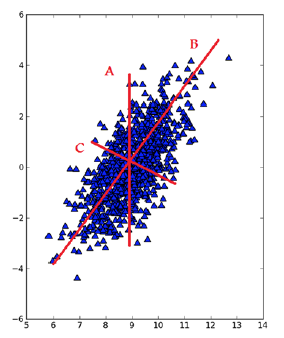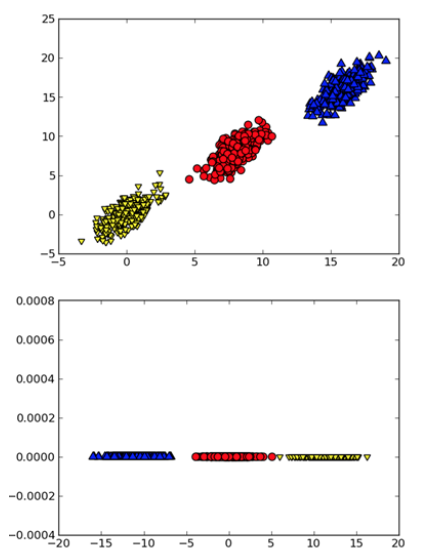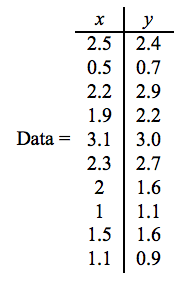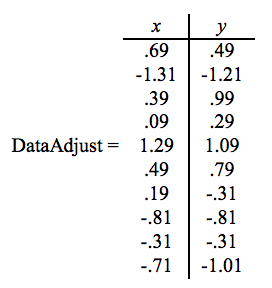# Principal Component Analysis (PCA)

## 1 PCA简介

1、拿到一个汽车的样本，里面既有以“千米/小时”度量的最大速度特征，也有“英里/小时”的最大速度特征，显然这两个特征有一个多余。
2、拿到一个数学系的本科生期末考试成绩单，里面有三列，一列是对数学的兴趣程度，一列是复习时间，还有一列是考试成绩。我们知道要学好数学，需要有浓厚的兴趣，所以第二项与第一项强相关，第三项和第二项也是强相关。那是不是可以合并第一项和第二项呢？

PCA的基本思想是 将 $$n$$ 维特征映射到 $$k \; (k < n)$$ 维上，这 $$k$$ 维是全新的正交特征。这 $$k$$ 维特征称为主元，是重新构造出来的 $$k$$ 维特征，而不是简单地从 $$n$$ 维特征中去除其余 $$n-k$$ 维特征。

## 2 PCA基本原理及步骤

PCA的基本步骤如下：
(1) 对所有训练数据都减去均值。
(2) 计算协方差矩阵
(3) 计算协方差矩阵的特征值和特征向量
(4) 将特征值从大到小排序，保留最前面的 $$k$$ 个特征值对应的特征向量
(5) 将数据转换到上述 $$k$$ 个特征向量构建的新空间中，得到最后降维的数据。

PCA的基本原理如下：Figure 1: 直线B是数据集中差异化最大的方向，选择它为第一坐标轴；和B正交的C是第二条坐标轴Figure 2: 二维空间的3个类别。应用PCA可对数据降维（本例中可以删除一个维度），从而分类更容易处理

PCA主成分分析Python实现：http://blog.csdn.net/Dream_angel_Z/article/details/50760130

### 2.1 PCA简单实例Figure 3: 原始数据（共二维）Figure 4: 所有数据都减去均值后的数据

$cov = \begin{pmatrix} 0.616555556 & 0.615444444 \\ 0.615444444 & 0.716555556 \\ \end{pmatrix}$

$eigenvalues = \begin{pmatrix} 0.0490833989 \\ 1.28402771 \\ \end{pmatrix}$
$eigenvectors = \begin{pmatrix} eig_1 & eig_2 \end{pmatrix} = \begin{pmatrix} -0.735178656 & -0.677873399 \\ 0.677873399 & -0.735178656 \\ \end{pmatrix}$

$FinalData_{m \times k} = DataAdjust_{m \times n} \times EigenVectors_{n \times k}$

\begin{aligned} FinalData & = \begin{pmatrix} 0.69 & 0.49 \\ -1.31 & -1.21 \\ 0.39 & 0.99 \\ 0.09 & 0.29 \\ 1.29 & 1.09 \\ 0.49 & 0.79 \\ 0.19 & -1.31 \\ -1.81 & -0.81 \\ -0.31 & -0.31 \\ -0.71 & -1.01 \end{pmatrix} \begin{pmatrix} -0.677873399 \\ -0.735178656 \end{pmatrix} \\ & = \begin{pmatrix} -0.827970186 \\ 1.77758033 \\ -0.992197494 \\ -0.274210416 \\ -1.67580142 \\ -0.912949103 \\ 0.0991094375 \\ 1.14457216 \\ 0.438046137 \\ 1.22382056 \end{pmatrix} \\ \end{aligned}

A tutorial on Principal Components Analysis: http://www.cs.otago.ac.nz/cosc453/student_tutorials/principal_components.pdf

### 2.2 更多例子

PCA的更多例子可参考下面资料：
《机器实习实战》13.3 示例：利用PCA对半导体制造数据降维
《机器学习：实用案例解析》第8章 PCA：构建股票市场指数

## 3 PCA的特点

PCA技术的一个很大的优点是，它是完全无参数限制的。在PCA的计算过程中完全不需要人为的设定参数或是根据任何经验模型对计算进行干预，最后的结果只与数据相关，与用户是独立的。

Created: <2015-12-29 Tue 00:00>

Last updated: <2017-11-22 Wed 21:53>

Creator: Emacs 25.3.1 (Org mode 9.1.2)